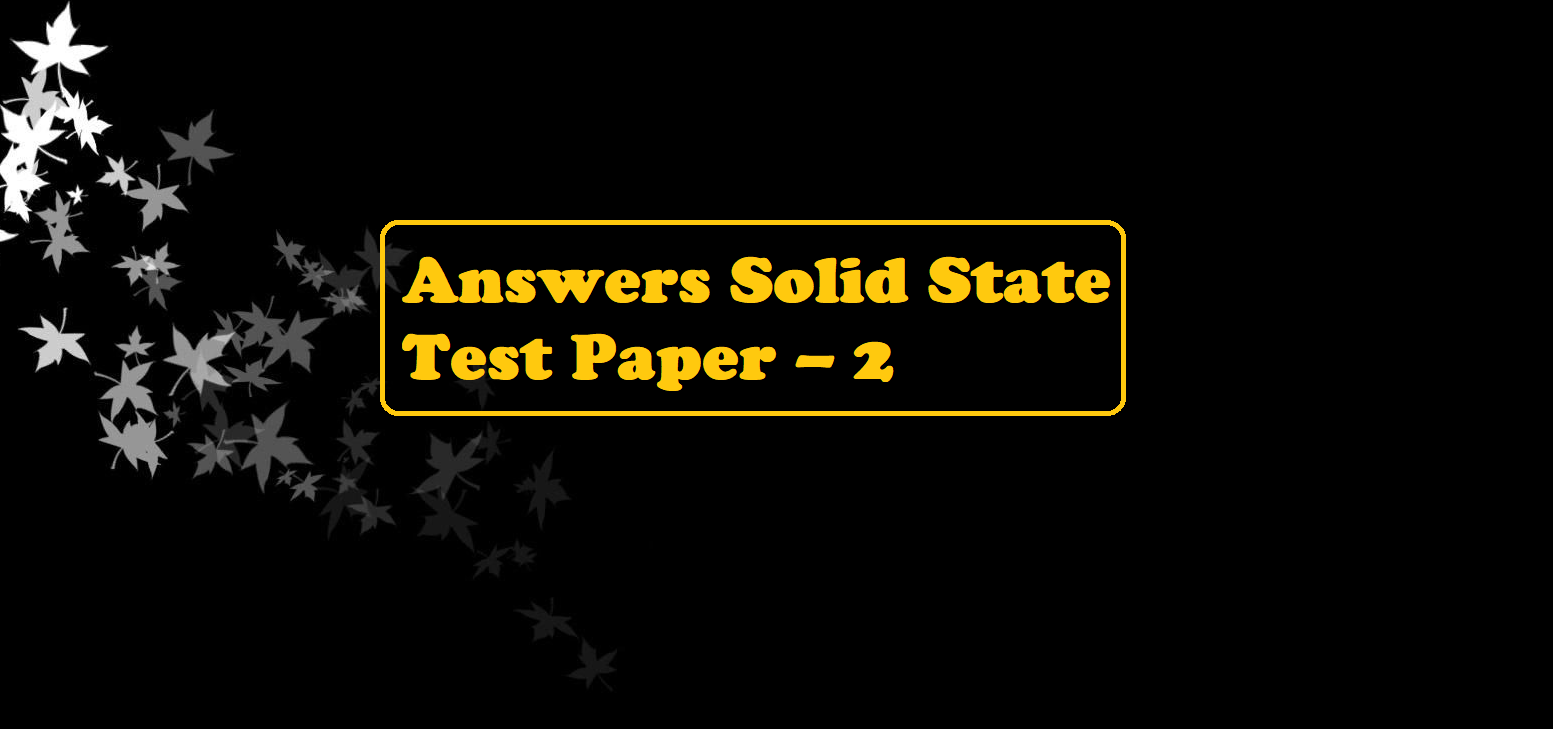# Answers Solid State Test Paper – 2To get Practice Questions click the post Solid State Test Paper – 2

# Answers Solid State Test Paper – 2

Answers Solid State Test Paper – 2

1. (B) 8

2. (B) Covalent

3. (A) Ionic solids

4. (A) Clion form fcc lattice and Na+ ions occupy all octahedral voids of the unit cell.

5. (C) 4

6. (A) Frenkel defect

7. We know for fcc,
2r+ + 2r = a
2(110) + 2r = 508 Þ 220 + 2r = 508
2r = 508 – 220 = 288
r = 144pm

8. Let a be the edge length and r be the radius of an atom9. (A) Zinc ions occupy half of the tetrahedral sites

10. (A) Cations and anions have almost equal size

11. (B) fcc> bcc > simple cubic

12. (B) high pressure

13. (D) KCl

14. (C) 4 and 8

15. (D) SrCl2

16. (D) n-type semiconductor

17. (A) X4Y6

18. (A) Vacancy defect

19. (A) Edge centers and along diagonals

20. (C) a = b = c and α = β = γ = 90

21. Coordination number of Na+ ion = 6

Coordination number of Cl ion = 6

Answers Solid State Test Paper – 2

22.23. We know 2 mg = 0.002 g
197g of Gold contains gold atoms = 6.022 × 1023
1g of Gold contains gold atoms = 6.022 × 1023 / 197
0.002 g of Gold contains gold atoms = (6.022 × 1023 / 197) 0.002
= 6.1137 × 1018 gold atoms.
1 unit cell of fcc lattice contains atoms. = 4
The number of unit cell in fcc = 6.1137 × 1018 / 4
=1.5 × 1018 unit cells

24. Atoms P per formula cell = 8 × 1 / 8 = 1 8×1/8=1
Atoms Q per unit cell =1,
Atoms R per unit cell =1,
Atoms R per unit cell = 6 × 1 / 2 = 3 6 × ½ = 3
Hence the formula is PQR3

25. In ZnO,
ZnO → Zn2+ + 1/2O2 + 2e
Zn2+ ions occupy the interstitial sites and electrons are trapped in the interstitial sites for neutralization. Crystals that show such types of defects are generally colored. Zinc oxide is white in color but on heating, it turns yellow because it loses oxygen molecules at high temperatures.

26. Atoms of X are present at all the eight corners of the cube. Therefore, each atom of X at the corner makes a 1/8 contribution towards the unit cell.
Number of atoms of X per unit cell = 8 × 1/8 = 1
Y atom is present at the body center, thus the contribution of Y towards unit cell = 1 × 1 = 1
O atom is present at each of the edge centers (number of edges of cube = 12)
And each O atom present at the edge center will make a 1/4 contribution towards the unit cell.
The number of O atoms per unit cell = 12 × ¼ = 3
The formula of the compound is, therefore XYO3

27. (a) In a Primitive cubic lattice, each atom is in direct contact with six other atoms. So the coordination number of the primitive cubic lattices is 6.

(b) The face-centered cubic (fcc) has a coordination number of 12 and contains 4 atoms per unit cell.
(c) In a body-centered cubic lattice, each atom is in direct contact with eight other atoms, So the coordination number of the body-centered cubic lattice is 8.

28. Given
Unit cube length of LiCl = 5.14 Å29. Gold occupies the corner of the unit cell.
There are 8 corners and contribution of each corner = 1/8
Therefore, total gold atom = 8 × 1/8 = 1
Copper occupies the faces of cubes. There are 6 faces and the contribution of each face is 1/2​
Therefore, the Total atoms on faces are = 6 × ½ = 3
Hence the formula is AuCu3

30. Oxidation no. of Fe in wurtzite is 200/93 = 2.15.
It is an intermediate value between two oxidation states of Fe, Fe (II) and Fe(III)
Let the percentage of Fe (III) be, then
2 × (100 – ∝) + 3 × ∝  = 2.15 × 100
∝ = 15
∴ Percentage of Fe(III) = 15%

Answers Solid State Test Paper – 2